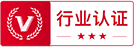QQ群反馈# 从零开始做AE表达式动画之——Virus落水弹跳动画

1665 24 17 4
2020-04-01
12.7*病毒落水的弹跳，水位上升的弹跳均用了同一段表达式

numKeys：关键帧的数量，返回值是一个整数，比如你在某属性上打了两个关键帧，该属性下的numKeys=2。nearestKey(time).index：先翻译下，“最近的关键帧（时间）.索引”，“.”在函数里通常可以翻译成“的”，脑补一下就是“离时间线指针最近的关键帧的的索引（序号）”，返回值是一个整数。key(n).time：第n个关键帧所在的时间，输入n的值，输出时间的值，单位默认是秒。比如在一个属性中，打下两个关键帧，第2个关键帧所在的时间是3秒，key(2).time=3。velocityAtTime：在某时的速度，这句几乎不需要想象力。

```amp = .1;
freq = 2.0;
decay = 2.0;
n = 0;
if (numKeys > 0){
n = nearestKey(time).index;
if (key(n).time > time){n--;}
}
if (n == 0){ t = 0;}
else{t = time - key(n).time;}
if (n > 0){
v = velocityAtTime(key(n).time - thisComp.frameDuration/10);
value + v*amp*Math.sin(freq*t*2*Math.PI)/Math.exp(decay*t);
}
else{value}```

amp = .1;//定义一个叫amp（振幅）的变量，赋值0.1

freq = 2.0;//定义一个叫freq（频率）的变量，赋值2.0

decay = 2.0;//定义一个叫decay（衰减）的变量，赋值2.0

n = 0;//定义一个叫n的变量，初始值为0，具体用来干嘛，继续往下看

n = nearestKey(time).index;//马上就告诉你，n默认表示离时间线最近的关键帧的序号，本质是一个数字

t = time - key(n).time；//t是时间线与某个关键帧所在时间的差值

thisComp.frameDuration/10//此合成帧时长的十分之一，如果一个合成共100帧，时长5秒，则thisComp.frameDuration/10=5/100/10=0.005

v = velocityAtTime(key(n).time - thisComp.frameDuration/10);//还用上一句注释中的例子，v就表示在某序号为n的关键帧前0.005秒时的速度

value + v*amp*Math.sin(freq*t*2*Math.PI)/Math.exp(decay*t);//So，将表达式梳理成流程图之后如下：1.当无关键帧时，numKeys=0，开始判断n是否为0，在一开始的定义中，n=0，故最后t=0，n当然也不大于0，最后执行value值，也就是属性本身的值。流程走向如下图高亮部分：2.1.当numKey=1的时候，如下图情形，有一个关键帧，时间线在关键帧之前或者之后。当时间线在关键帧之前时，key(1).time>time,n—(即n=n-1)，n=1-1=0,所以t=0…最后执行value。2.2.当时间线在关键帧之后时，key(1).time

“v = velocityAtTime(key(1).time - thisComp.frameDuration/10);

value + v*amp*Math.sin(freq*t*2*Math.PI)/Math.exp(decay*t);“图9（关键帧为1 且时间线在关键帧前后 流程图）

3.在本文病毒落水的动画中，关键帧数量为2，即numKey=2，时间线的位置有以下几种可能:

A.位于第一个关键帧之前（key(1).time>time,n=n-1）

B.位于两个关键帧之间但离第一个更近（key(1).time

C.位于两个关键帧之间但离第二个更近（key(2).time>time,n=n-1）

D.位于第二个关键帧后面(key(2).time```amp = .1;
freq = 2.0;
decay = 2.0;
n = 0;
if (numKeys > 0){
n = nearestKey(time).index;
if (key(n).time > time){n--;}
if (n <= 0){value}
else{
t = time - key(n).time;
v = velocityAtTime(key(n).time - thisComp.frameDuration/10);
value + v*amp*Math.sin(freq*t*2*Math.PI)/Math.exp(decay*t);}
}
else{value}``````amp=0.1;
freq=10;
decay=2;
a=numKeys;
n=nearestKey(time).index;
et=key(n).time;
t=time-et;
v=velocityAtTime(et-0.01);
if  (t>0 & n>=a){
value+v*amp*Math.sin(t*freq)/Math.exp(t*decay);
}
else{
value;
}```

“if  (t>0 & n>=a){

value+v*amp*Math.sin(t*freq)/Math.exp(t*decay);

}

t>0即时间线在离它最近的关键帧后面；且n>=a,即n大于关键帧的总个数；当两个条件均满足的时候，表示时间线在最后一个关键帧后面，此时开始绘制弹性曲线，否则就走value值。是不是非常简单，非常直接，非常清爽？简单到画流程图都是多余！我只是想在动画的末尾加上一丢丢弹性啊，这样就很完美了不是么？# Hicy

1885粉丝/53关注0/200

• 全部设计师
• 推荐设计师和认证设计师

### 您确认要推荐？

#### 推荐心得

0/200//